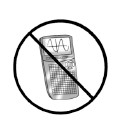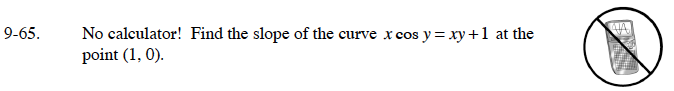### Home > CALC > Chapter 9 > Lesson 9.2.2 > Problem9-65

9-65.

No calculator! Find the slope of the curve x cos y = xy + 1 at the point (1, 0). Homework Help ✎$\frac{d}{dx}xcos(y)=\frac{d}{dx}\Big(xy+1)\Big)$

$cos(y)-xsin(y)y^\prime=y+xy^\prime$

Solve for y′.

Evaluate the equation from step 2 for x = 1 and y = 0.